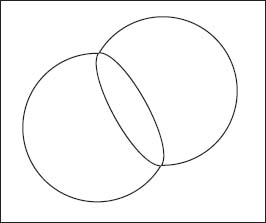NextPrevious

# Have computers been used to solve mathematical proofs?

Yes, there have been many mathematical proofs solved with the help of computers. One example is the four color theorem, which stated that it is possible to have a geographic map colored with only four colors so that no adjacent regions will have the same color. Another way of looking at the problem is: What is the smallest number of colors needed to color any flat map so that any two neighboring regions always have different colors? This idea was first presented in 1852, when Francis Guthrie (1831–1899) colored a map of English counties using only four colors. The idea of only four colors took on a mathematical bend and ended up being a theorem to be proved. It took until 1976, with the help of modern computers, before the four-color conjecture was finally proven to be true. Wolfgang Haker (1928-) and Kenneth Appel (1932-) of the University of Illinois took four years to write the computer program for the Cray computer, which took 1,200 hours to check 1,476 configurations. And in 2005, it was proven by Georges Gonthier with general purpose theorem-proving software.

Even though the theorem-proving software allows calculations to be checked along the way (by the computer), not all mathematicians are content with the result. They are troubled by the fact that the theorem was still proven by a computer, feeling that if it’s so easy to understand it should have been proven by hand. Thus, anyone who can truly prove the theorem without using a computer may win the Fields Medal, the math equivalent of the Nobel Prize.

Another proof solved with computers is the double bubble. The double bubble refers to a pair of bubbles that intersect; they are also separated by a membrane bounded by the intersection of the two bubbles. This is similar to two bubbles stuck together when a child blows bubbles using a water and soap mixture. Since the ancient Greeks, mathematicians have worked on the problem of finding a mathematical proof of the efficiency of a single round bubble. The problem became even more rigorous when considering enclosing two bubbles—or two separate volumes. The problem was solved around 1995 by mathematicians Joel Hass, Michael Hutchings, and Roger Schlafly. They used a computer to calculate the surface areas of the bubbles and found that the double bubble has a smaller area than any other when the enclosed volumes are the same. But this isn’t the last word: Scientists are currently working on triple bubbles.In the problem of the double bubble, ancient Greek mathematicians worked on a proof that would demonstrate the efficient use of space created by two joined bubbles.

Close

This is a web preview of the "The Handy Math Answer Book" app. Many features only work on your mobile device. If you like what you see, we hope you will consider buying. Get the App